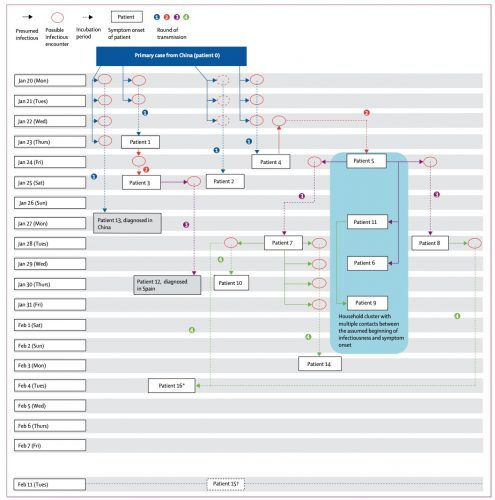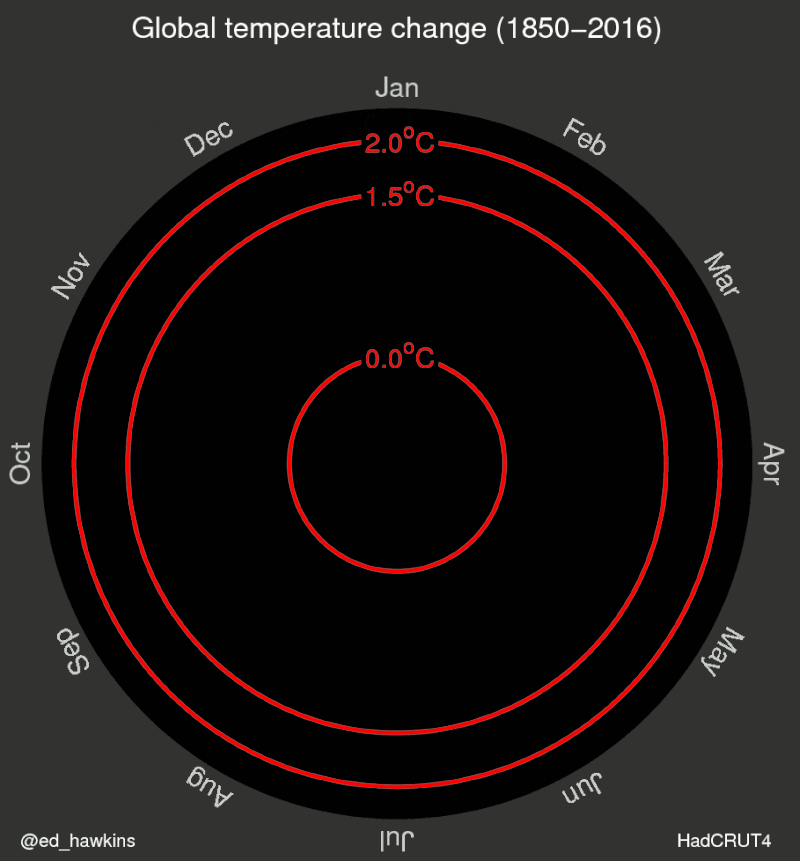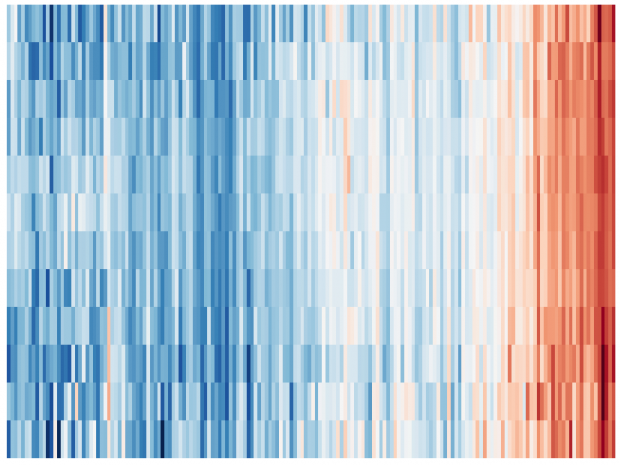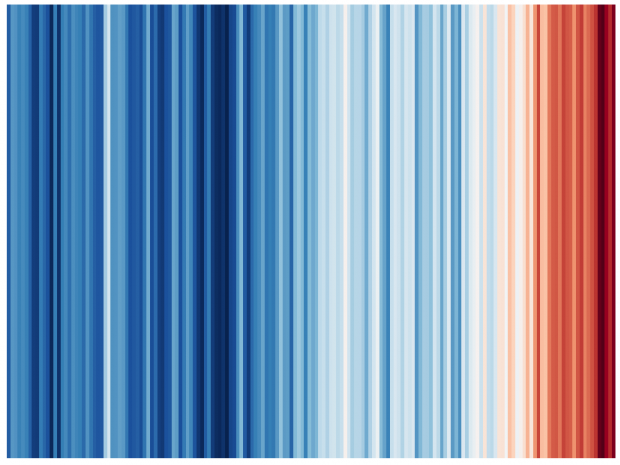# Graphical display of outbreaks: Transmission trees

Let’s start with some examples from the literature, find out necessary elements, compare different versions and develop a R template for general use.

“Exploding Stars”

“Tetris”

“Clean Undeterministic”
“Clean Tree”

“Railways”

“Simple ”

“Vertical”“star bus”

“Mathematical”

“Genetics”

# From R to Python

It’s bit confusing if you are having long-term experience with R but need some OpenCV Python code. What worked for me

2. pip install opencv-python
3. pip install opencv-contrib-python
4. although Spyder or Jupyter is recommended for data science, I went for PyCharm
5. install Atom and follow the video instructions
6. take care, numerous non working introductions out there, stick to recent version

# R leaflet – keep map in frame after closing info box

Although having some experience with leaflet before, it took me 5 hours to find out how to
(1) change the background color and
(2) re-center my map after the popup was panning the map  basically out view. Here is my solution

```leaflet(options = leafletOptions(
zoomControl = FALSE,
minZoom=6, maxZoom=6,
centerFixed = TRUE) ) %>%
fillColor = ~pal(AnzahlFall),
color="black", weight = 1,
fillOpacity = 0.7,
label = ~name_2,
popup = ~www,
popupOptions = popupOptions( autopan=FALSE, keepInView = TRUE ) ) %>%
htmlwidgets::onRender("
function(el, x) {
var myMap = this;
myMap.dragging.disable();
myMap.on('popupclose', function(e) { myMap.panTo( {lon: 10.26, lat: 51.1} ) });
var e = document.getElementsByClassName('leaflet-container');
e.style.backgroundColor = 'white';
}")
```

# A new animation of the famous HadCRUT4 climate dataset

Here is the R sample code (PPT aspect ratio is 6:4, Youtube wants 16:9) .

As ggplot2 animation packages have major difficulties to manipulate the single frames, I am combining here raw PNGs using ffmpeg.

```# read_cru_hemi() modified from https://mccartneytaylor.com/plotting-climate-change-on-a-spider-graph-using-r

list.of.packages <- c("ggplot2", "reshape", "stringr","RColorBrewer")
new.packages <- list.of.packages[!(list.of.packages %in% installed.packages()[,"Package"])]
if(length(new.packages)) install.packages(new.packages)
lapply(list.of.packages, require, character.only = TRUE)

nrows <- nrow(tab)
hemi <- data.frame(
year=tab[seq(1,nrows,2),1],
annual=tab[seq(1,nrows,2),14],
month=array(tab[seq(1,nrows,2),2:13]),
cover=array(tab[seq(2,nrows,2),2:13])
)
hemi[,15:26][ hemi[,15:26]==0 ] <- c(NA)
return(hemi)
}

tempmelt <- melt(tempdat[,c(1,3:14)],id="year")

colfunc <- colorRampPalette(c("grey","grey","red"))

new_theme <- theme_classic() + theme(
text = element_text(size=18, colour="grey"),
axis.line = element_blank(),
axis.text = element_text(colour="grey"),
axis.ticks = element_line(colour="grey"),
axis.title.x = element_blank(),
panel.border = element_blank(),
panel.grid.major = element_blank(),
panel.grid.minor = element_blank(),
panel.background = element_blank(),
legend.position = "none"
)
theme_set(new_theme)

for(i in 1850:2019){
p <- ggplot(tempmelt[tempmelt\$year %in% 1850:i,], aes(x=variable,y=value,color=as.factor(year),group=year)) +
geom_line() +
scale_x_discrete( labels=month.abb) +
scale_y_continuous( name="difference from baseline  [ oC ]", limits=c(-1,1) ) +
annotate("text", x=11, y=1, label=i, size=7) +
ggsave(p, file=fn, width = 16, height = 9)
}

# not run
# ffmpeg -framerate 10 -i /Users/xxx/Desktop/X/%3d.png -r 5 -pix_fmt yuv420p -y /Users/xxx/Desktop/X/out.mp4
```

In comparison here is the original circular plot. Would require blue, green, yellow, red in the Color Ramp Palette…Now it is only a minor step to the warming strips.

```ggplot(tempdat, aes(x = year, y = 1, fill = annual))+
geom_tile()+
scale_y_continuous(expand = c(0, 0))+
scale_x_continuous(expand = c(0, 0))+
guides(fill = guide_colorbar(barwidth = 1)) +
theme( axis.ticks= element_blank(),
axis.text = element_blank(),
axis.title = element_blank()
)

tempmelt\$variable <- as.numeric(str_replace(as.character(tempmelt\$variable),"month.",""))
ggplot(tempmelt, aes(x = year, y = variable, z = value)) +
geom_raster(aes(fill = value)) +
scale_x_continuous(expand = c(0,0)) +
scale_y_continuous(expand = c(0,0)) +
theme( axis.ticks= element_blank(),
axis.text = element_blank(),
axis.title = element_blank()
)
```# Rstudio, knitr (Rmarkdown2) and bash

I couldn’t find any example online how to revise my R code getting the exif data from pictures

```fn <- c("/usr/local/bin/exiftool /Users/wjst/Desktop/white.tif")
info <- system(fn,inter=TRUE,wait=TRUE)
```

when moving now to knitr. So here is what worked for me as a replacement including the parsing
of exiftool output.

``````{r, engine='bash', echo=FALSE}
/usr/local/bin/exiftool /Users/wjst/Desktop/white.tif >/Users/wjst/Desktop/white.txt
```
```{r Exif, echo=FALSE}
fn <- '/Users/wjst/Desktop/white.txt'
info <- strsplit(info,"[:]{1}[ ]{1}")
info <- matrix(data=unlist(info), ncol = 2, byrow = TRUE)
info <- gsub("(^[[:space:]]+|[[:space:]]+\$)", "", info)
```
*Exif*
`r kable(info)`
```

# Better figures

A recent paper identifies 10 rules for better pictures. As I have also given several lectures on that topic, I was excited what the authors think…
1. Know your audience. This is trivial as you never know your audience.
2. Identify your message. True and not true at the same time. True as it makes your findings more evident – not true if you are allowing a reader to find his own message.
3. Adapt the figure to the support medium. Trivial. May be very time consuming.
4. Captions are not optional. Absolutely true, I also suppport good captions – mini stories for those who can’t read the whole text.
5. Do not trust the defaults. Trivial. No one does.
6. Use color efficiently.  Not really,  avoid colors for those of us who are colorblind and to avoid expensive page charges.